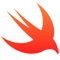/ Framework

# xxHash framework in Swift## xxHash-Swift

Framework include xxHash 32/64 bit functions.

Original xxHash algorithm created by Yann Collet.

# Install

## Carthage

`github "daisuke-t-jp/xxHash-Swift"`

## CocoaPods

``````use_frameworks!

target 'target' do
pod 'xxHash-Swift'
end
``````

# Usage

## Import framework

``````import xxHash_Swift
``````

## Generate Hash(One-shot)

### 32bit Version

``````let hash = xxHash32.digest("123456789ABCDEF")
// hash -> 0x576e3cf9

// Using seed.
let hash = xxHash32.digest("123456789ABCDEF", seed: 0x7fffffff)
// hash -> 0xa7f06f9d
``````

### 64bit Version

``````let hash = xxHash64.digest("123456789ABCDEF")
// hash -> 0xa66df83f00e9202d

// Using seed.
let hash = xxHash64.digest("123456789ABCDEF", seed: 0x000000007fffffff)
// hash -> 0xe8d84202a16e482f
``````

## Generate Hash(Streaming)

### 32bit Version

``````// Create xxHash instance
let xxh = xxHash32() // if using seed, e.g. "xxHash(0x7fffffff)"

// Get data from file
let bundle = Bundle(for: type(of: self))
let path = bundle.path(forResource: "alice29", ofType: "txt")!
let data = NSData(contentsOfFile: path)
let array = [UInt8](data! as Data)

let bufSize = 1024
var index = 0
var flag = true

repeat {
// Prepare buffer
var buf = [UInt8]()
for _ in 0..<bufSize {
buf.append(array[index])
index += 1
if index >= array.count {
flag = false
break
}
}

// xxHash update
xxh.update(buf)

} while(flag)

let hash = xxh.digest()
// hash -> 0xafc8e0c2
``````

### 64bit Version

``````// Create xxHash instance
let xxh = xxHash64() // if using seed, e.g. "xxHash(0x0000007fffffff)"

// Get data from file
let bundle = Bundle(for: type(of: self))
let path = bundle.path(forResource: "alice29", ofType: "txt")!
let data = NSData(contentsOfFile: path)
let array = [UInt8](data! as Data)

let bufSize = 1024
var index = 0
var flag = true

repeat {
// Prepare buffer
var buf = [UInt8]()
for _ in 0..<bufSize {
buf.append(array[index])
index += 1
if index >= array.count {
flag = false
break
}
}

// xxHash update
xxh.update(buf)

} while(flag)

let hash = xxh.digest()
// hash -> 0x843c2c4ccfbfb749
``````

## GitHub#### iOS Example

A nice collection of often useful examples done in Objective-C and Swift.

Latest
Tag